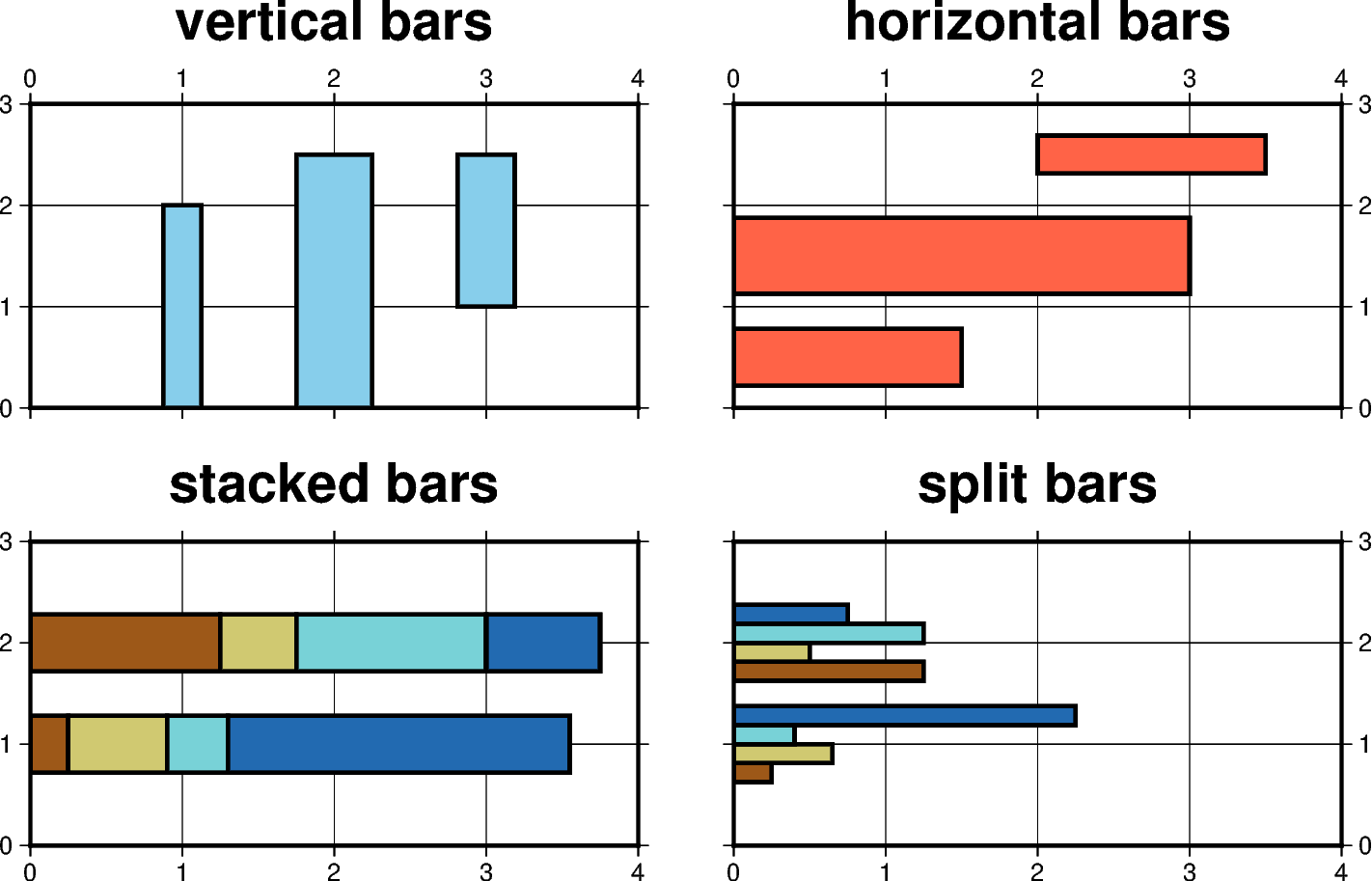# Vertical or horizontal bars

The `pygmt.Figure.plot` method can plot vertical (b) or horizontal (B) bars by passing the corresponding shortcut to the `style` parameter. By default, base = 0 meaning that the bar is starting from 0. Append +b[base] to change this value. To plot multi-band bars, please append +v|iny (for verticals bars) or +v|inx (for horizontal ones), where ny or nx indicate the total number of bands in the bar (and hence the number of values required to follow the x,y coordinate pair in the input). Here, +i means we must accumulate the bar values from the increments dy or dx, while +v means we get the complete values relative to base. Normally, the bands are plotted as sections of a final single bar. Use +s to instead split the bar into ny or nx side-by-side, individual and thinner bars. Multi-band bars require `cmap=True` with one color per band.```import pygmt

fig = pygmt.Figure()

pygmt.makecpt(cmap="roma", series=[0, 4, 1])

with fig.subplot(
nrows=2,
ncols=2,
subsize=("8c", "4c"),
frame="ag",
sharey=True,
sharex=True,
margins=["0.5c", "0.75c"],
):
pen = "1.5p"
with fig.set_panel(panel=0):
fill = "skyblue"
fig.basemap(region=[0, 4, 0, 3], frame="+tvertical bars")
fig.plot(x=1, y=2, style="b0.5c", fill=fill, pen=pen)
fig.plot(x=2, y=2.5, style="b1c", fill=fill, pen=pen)
# +b1 means that the bar is starting from y=1 here
fig.plot(x=3, y=2.5, style="b0.75c+b1", fill=fill, pen=pen)

with fig.set_panel(panel=1):
fill = "tomato"
fig.basemap(region=[0, 4, 0, 3], frame="+thorizontal bars")
fig.plot(x=1.5, y=0.5, style="B0.75c", fill=fill, pen=pen)
fig.plot(x=3, y=1.5, style="B1c", fill=fill, pen=pen)
# +b2 means that the bar is starting from x=2 here
fig.plot(x=3.5, y=2.5, style="B0.5c+b2", fill=fill, pen=pen)

# generate dictionary for plotting multi-band bars
data = {
"x1": [0.25, 1.25],
"y": [1, 2],
"x2": [0.65, 0.5],
"x3": [0.4, 1.25],
"x4": [2.25, 0.75],
}

with fig.set_panel(panel=2):
fig.basemap(region=[0, 4, 0, 3], frame="+tstacked bars")
fig.plot(data=data, style="B0.75c+i4", cmap=True, pen=pen)

with fig.set_panel(panel=3):
fig.basemap(region=[0, 4, 0, 3], frame="+tsplit bars")
fig.plot(data=data, style="B1c+v4+s", cmap=True, pen=pen)

fig.show()
```

Total running time of the script: ( 0 minutes 2.146 seconds)

Gallery generated by Sphinx-Gallery